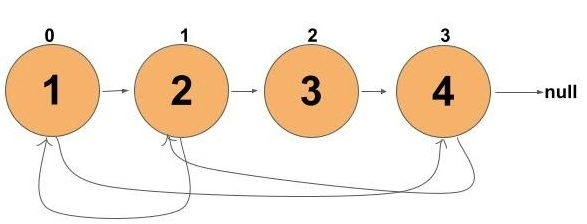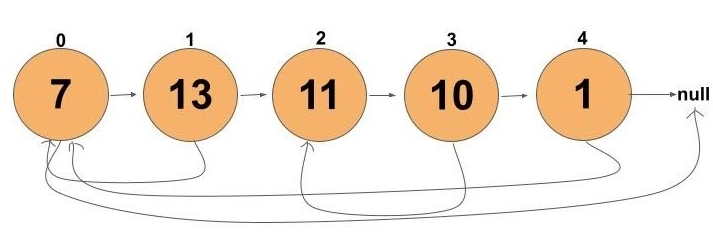# Clone Linked List with Random and Next Pointer

Problem Statement: Given a Linked list that has two pointers in each node and one of which points to the first node and the other points to any random node. Write a program to clone the LinkedList.

```Example 1:
Input:
Output:
Explanation:```
```Example 2:
Input:
Output:

Explanation:```

## Solution 1: Brute Force

### Approach:

We will use a hash-map for keeping track of deep copies of every node.

• Iterate through the entire list.
• For each node, create a deep copy of each node and hash it with it. Store it in the hashmap.
• Now, again iterate through the given list. For each node, link the deep node present as the hash value of the original node as per original node.
• the head of the deep copy list will be the head of hashed value of original node.

### Dry Run:

We will iterate through the list. For each node, we will create its deep copy node and hash it with the current node.

(Not to confuse with new nodes created in the hash table, these nodes are represented by (‘))

Now we will iterate through the original list again. Since deep copies of each node are hashed with the original node, we can access from original nodes and link them.

Hence, we achieved the deep copy of the original linked list accessible by the hashed value head of the original list.

## C++ Code

``````#include<bits/stdc++.h>
using namespace std;

class Node {
public:
int val;
Node* next;
Node* random;
Node(int value) {
val = value;
next = NULL;
random = NULL;
}
};

unordered_map<Node*,Node*> hashMap;
//first iteration for inserting deep nodes of every node in the hashmap.
while(temp != NULL) {
Node* newNode = new Node(temp->val);
hashMap[temp] = newNode;
temp = temp->next;
}
//second iteration for linking next and random pointer as given question.
while(t != NULL) {
Node* node = hashMap[t];
node->next = (t->next != NULL) ? hashMap[t->next]:NULL;
node->random = (t->random != NULL) ? hashMap[t->random]:NULL;
t = t->next;
}
}

cout<<endl;
}
}

int main() {

Node* node1 = new Node(1);
Node* node2 = new Node(2);
Node* node3 = new Node(3);
Node* node4 = new Node(4);

cout<<"Original list:(current node:node pointed by next pointer,node pointed
by random pointer)\n";

cout<<"Copy list:(current node:node pointed by next pointer,node pointed by
random pointer)\n";
return 0;
}
``````

Output:

Original list:(current node: node pointed by next pointer, node pointed by random pointer)
1:2,4
2:3,1
3:4, NULL
4:NULL,2
Copy list:(current node: node pointed by next pointer, node pointed by random pointer)
1:2,4
2:3,1
3:4, NULL
4:NULL,2

Time Complexity: O(N)+O(N)

Reason: Two iterations over the entire list. Once for inserting in the map and other for linking nodes with next and random pointer.

Space Complexity: O(N)

Reason: Use of hashmap for storing entire data.

## Java Code

``````import java.util.*;

class Node {
int val;
Node next;
Node random;
Node(int value) {
val = value;
next = null;
random = null;
}
}
class TUF{
HashMap<Node,Node> hashMap=new HashMap<>();
//first iteration for inserting deep nodes of every node in the hashmap.
while(temp != null) {
Node newNode = new Node(temp.val);
hashMap.put(temp,newNode);
temp = temp.next;
}
//second iteration for linking next and random pointer as given question.
while(t != null) {
Node node = hashMap.get(t);
node.next = (t.next != null) ? hashMap.get(t.next):null;
node.random = (t.random != null) ? hashMap.get(t.random):null;
t = t.next;
}
}

else
System.out.print("NULL");
else
System.out.print(",NULL");
System.out.println();
}
}

public static void main(String args[]) {

Node node1 = new Node(1);
Node node2 = new Node(2);
Node node3 = new Node(3);
Node node4 = new Node(4);

System.out.println("Original list:(current node:node pointed by next pointer,
node pointed by random pointer)");

System.out.println("Copy list:(current node:node pointed by next pointer,node
pointed by random pointer)");
}
}``````

Output:

Original list:(current node:node pointed by next pointer,node pointed by random pointer)
1:2,4
2:3,1
3:4, NULL
4:NULL,2
Copy list:(current node: node pointed by next pointer, node pointed by random pointer)
1:2,4
2:3,1
3:4, NULL
4:NULL,2

Time Complexity: O(N)+O(N)

Reason: Two iterations over the entire list. Once for inserting in the map and other for linking nodes with next and random pointer.

Space Complexity: O(N)

Reason: Use of hashmap for storing entire data.

## Solution 2: Optimized

### Approach:

The optimisation will be in removing the extra spaces, i.e, the hashmap used in brute force. This approach can be broken down into three steps.

• Create deep nodes of all nodes. Instead of storing these nodes in a hashmap, we will point it to the next of the original nodes.
• Take a pointer, say itr, point it to the head of the list. This will help us to point random pointers as per the original list. This can be achieved by itr->next->random = itr->random->next
• Use three pointers. One dummy node whose next node points to the first deep node. itr pointer at the head of the original list and fast which is two steps ahead of the itr. This will be used to separate the original linked list with the deep nodes list.

### Dry Run:

In brute force, we created new nodes and stored them in the hashmap. Here, we will create each node and link it next to the original nodes. This contributes to step1. (Blue colored nodes are deep copy nodes)

In step 2, we use a pointer itr. This pointer will help to create random pointer links among deep copy nodes. (Purple color lines show itr->next->random. Red color lines shows itr->random->next)

Since this node’s random pointer is pointing to NULL. Therefore, highlighted arrows are not present.

Our task is to differentiate the original list and deep copy one.

Finally returning dummy->next we get our desired answer.

Code:

## C++ Code

``````#include<bits/stdc++.h>
using namespace std;

class Node {
public:
int val;
Node* next;
Node* random;
Node(int value) {
val = value;
next = NULL;
random = NULL;
}
};

//step 1
while(temp != NULL) {
Node* newNode = new Node(temp->val);
newNode->next = temp->next;
temp->next = newNode;
temp = temp->next->next;
}
//step 2
while(itr != NULL) {
if(itr->random != NULL)
itr->next->random = itr->random->next;
itr = itr->next->next;
}
//step 3
Node* dummy = new Node(0);
temp = dummy;
Node* fast;
while(itr != NULL) {
fast = itr->next->next;
temp->next = itr->next;
itr->next = fast;
temp = temp->next;
itr = fast;
}
return dummy->next;

}

cout<<endl;
}
}

int main() {

Node* node1 = new Node(1);
Node* node2 = new Node(2);
Node* node3 = new Node(3);
Node* node4 = new Node(4);

cout<<"Original list:(current node:node pointed by next pointer,node pointed
by random pointer)\n";

cout<<"Copy list:(current node:node pointed by next pointer,node pointed by
random pointer)\n";
return 0;
}
``````

Output:

Original list:(current node:node pointed by next pointer,node pointed by random pointer)
1:2,4
2:3,1
3:4,NULL
4:NULL,2
Copy list:(current node: node pointed by next pointer, node pointed by random pointer)
1:2,4
2:3,1
3:4, NULL
4:NULL,2

Time Complexity: O(N)+O(N)+O(N)

Reason: Each step takes O(N) of time complexity.

Space Complexity: O(1)

Reason: No extra data structure was used for computation.

## Java Code

``````import java.util.*;

class Node {
int val;
Node next;
Node random;
Node(int value) {
val = value;
next = null;
random = null;
}
}
class TUF{
//step 1
while(temp != null) {
Node newNode = new Node(temp.val);
newNode.next = temp.next;
temp.next = newNode;
temp = temp.next.next;
}
//step 2
while(itr != null) {
if(itr.random != null)
itr.next.random = itr.random.next;
itr = itr.next.next;
}
//step 3
Node dummy = new Node(0);
temp = dummy;
Node fast;
while(itr != null) {
fast = itr.next.next;
temp.next = itr.next;
itr.next = fast;
temp = temp.next;
itr = fast;
}
return dummy.next;
}

else
System.out.print("NULL");
else
System.out.print(",NULL");
System.out.println();
}
}

public static void main(String args[]) {

Node node1 = new Node(1);
Node node2 = new Node(2);
Node node3 = new Node(3);
Node node4 = new Node(4);

System.out.println("Original list:(current node:node pointed by next pointer,
node pointed by random pointer)");

System.out.println("Copy list:(current node:node pointed by next pointer,node
pointed by random pointer)");
}
}``````

Output:

Original list:(current node: node pointed by next pointer, node pointed by random pointer)
1:2,4
2:3,1
3:4, NULL
4:NULL,2
Copy list:(current node: node pointed by next pointer, node pointed by random pointer)
1:2,4
2:3,1
3:4, NULL
4:NULL,2

Time Complexity: O(N)+O(N)+O(N)

Reason: Each step takes O(N) of time complexity.

Space Complexity: O(1)

Reason: No extra data structure was used for computation.